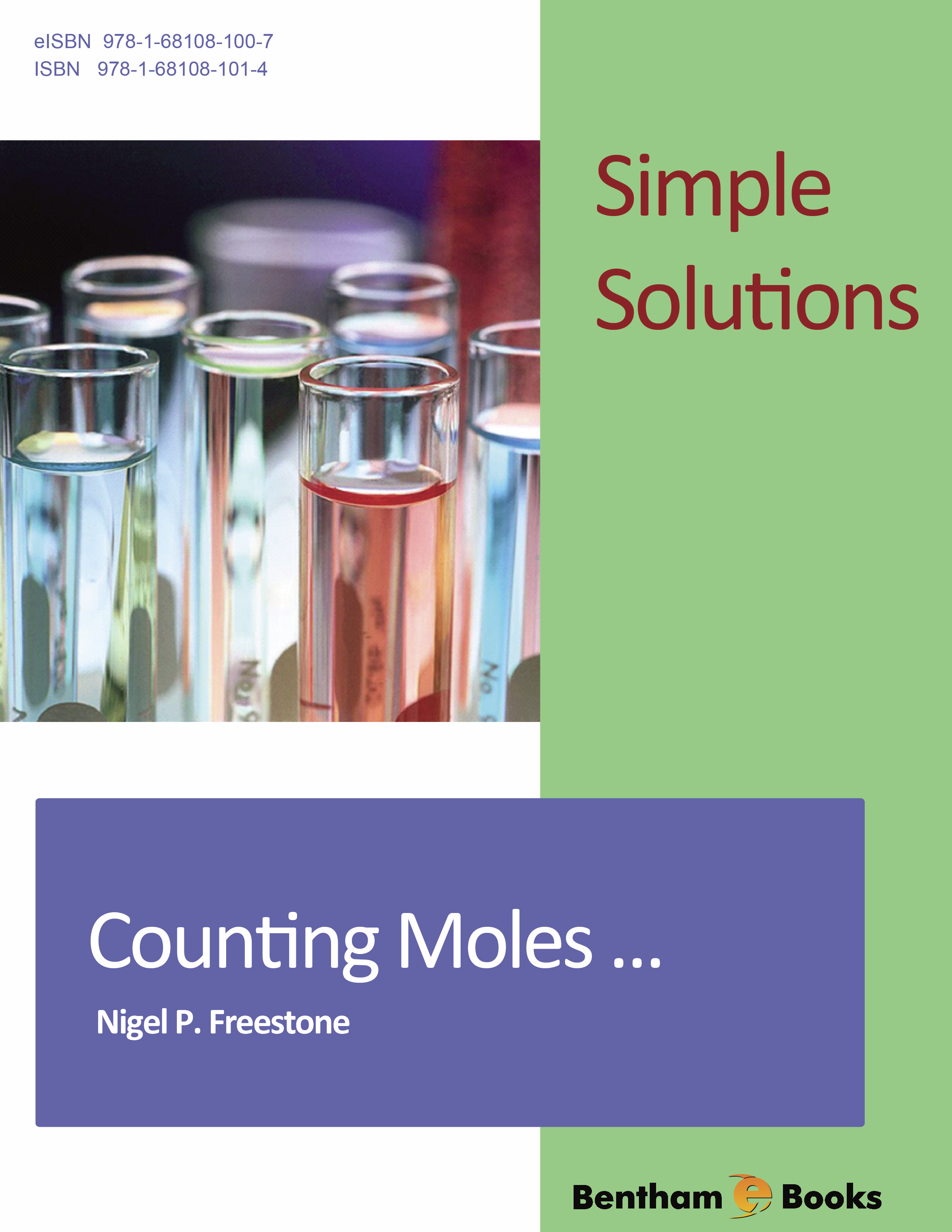Author: Nigel Freestone

### Simple Solutions in Chemistry – Counting Moles

eBook: US \$19 Special Offer (PDF + Printed Copy): US \$119
Printed Copy: US \$110
ISBN: 978-1-68108-101-4 (Print)
ISBN: 978-1-68108-100-7 (Online)
Year of Publication: 2016
DOI: 10.2174/97816810810071160101

#### Introduction

Students studying chemistry often struggle with the mole. Counting Moles provides an effective aid to learning by giving clear and confident presentation of the essentials of the mole concept needed by those starting chemistry courses. This user-friendly self-teach e-book is split into six chapters which sequentially introduce the ‘mole calculating frame’ to help solve problems. Over 200 fully worked examples are given along with several hundred questions.

The mole concept is applied to topics such as relative atomic mass and relative formula mass, percentage composition, empirical and molecular formula. The book also covers concentration, its units, volumetric analysis and the relationship between volume, mass and moles of gases. Counting Moles culminates in you taking a Mole Driving Test. On passing this test, you are issued with a Counting Moles Driving License that will give you all the confidence required to correctly answer all mole calculations.

Indexed in: EBSCO.

# Preface

Students studying chemistry often struggle with the MOLE. This user-friendly self-teach package provides an effective aid to learning by giving clear and confident presentation of the essentials of the mole needed by those starting chemistry courses. This self teach package contains over 200 questions, with detailed solutions, so if you get stuck, you can see where you went wrong.

After successfully completing Counting Moles, you will be able to:

• understand what is meant by the terms relative atomic mass and relative formula mass
• calculate average atomic mass from isotope abundance;
• define of the chemical term, the ‘mole’;
• calculate the molar mass of a substance from its chemical formula;
• calculate percentage composition;
• interconvert between mass, number of molecules, and moles;
• calculate the amount (mass or moles) of product expected to be formed in a chemical reaction, given the amounts of reactants used;
• calculate the amount (mass or moles) of reactants which need to be used in a chemical reaction in order to produce a specified amount of product;
• define the term concentration, and calculate the molarity of solutions from volumetric analysis and other data;
• determine the mass and/or volume of gases consumed or formed in a chemical reaction.

Counting Moles is split into SIX (6) Chapter. Each Chapter should be thought of as a separate lesson that should take between 15 and 45 minutes to complete. It is ESSENTIAL that you answer each question. The Counting Moles approach to learning is based on understanding developed through repetition and introduces the ‘mole calculating frame’ to help you solve problems. Answers to ALL questions, with full explanations are provided, should you get into difficulty.

The Chapters prepare you for the Counting Moles Driving Test. If you PASS, you can throw you M-plate away and claim your Counting Moles Driving Licence. You will then be able to answer moles-based coursework and examination questions correctly and with confidence.

None

#### Conflict of Interest

The author confirms that this book contents have no conflict of interest.